Standard 3-phase circuits or networks take on two major forms with names that represent the way in which the resistances are connected...

Standard 3-phase circuits or networks take on two major forms with names that represent the way in which the resistances are connected, a Star connected network which has the symbol of the letter, Υ (wye) and a Delta connected network which has the symbol of a triangle, Δ (delta).
If a 3-phase, 3-wire supply or even a 3-phase load is connected in one type of configuration, it can be easily transformed or changed it into an equivalent configuration of the other type by using either the Star Delta Transformation or Delta Star Transformation process.
A resistive network consisting of three impedances can be connected together to form a T or “Tee” configuration but the network can also be redrawn to form a Star or Υ type network as shown below.

### T-connected and Equivalent Star NetworkAs we have already seen, we can redraw the T resistor network above to produce an electrically equivalent Star or Υ type network. But we can also convert a Pi or π type resistor network into an electrically equivalent Delta or Δ type network as shown below.

### Pi-connected and Equivalent Delta Network.Having now defined exactly what is a Star and Delta connected network it is possible to transform the Υ into an equivalent Δ circuit and also to convert a Δ into an equivalent Υcircuit using a the transformation process. This process allows us to produce a mathematical relationship between the various resistors giving us a Star Delta Transformation as well as a Delta Star Transformation.
These circuit transformations allow us to change the three connected resistances (or impedances) by their equivalents measured between the terminals 1-2, 1-3 or 2-3 for either a star or delta connected circuit. However, the resulting networks are only equivalent for voltages and currents external to the star or delta networks, as internally the voltages and currents are different but each network will consume the same amount of power and have the same power factor to each other.

Three branches in an electrical network can be connected in numbers of forms but most common among them is either star or delta form. In delta connection, three branches are so connected, that they form a closed loop. As these three branches are connected nose to tail, they form a triangular closed loop, this configuration is referred as delta connection. On the other hand, when either terminal of three branches is connected to a common point to form a Y like pattern is known as star connection. But these star and delta connections can be transformed from one form to another. For simplifying complex network, delta to star or star to delta transformation is often required.

## Delta - Star Transformation

The replacement of delta or mesh by equivalent star connection is known as delta - star transformation. The two connections are equivalent or identical to each other if the impedance is measured between any pair of lines. That means, the value of impedance will be the same if it is measured between any pair of lines irrespective of whether the delta is connected between the lines or its equivalent star is connected between that lines.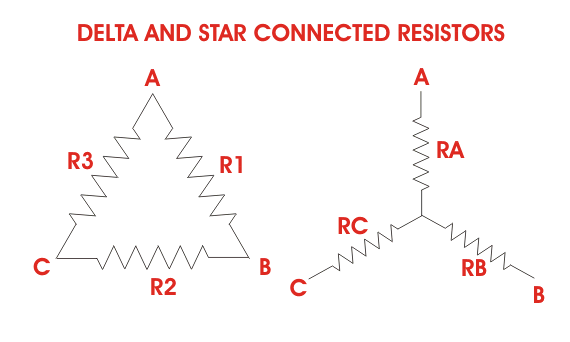Consider a delta system that's three corner points are A, B and C as shown in the figure. Electrical resistance of the branch between points A and B, B and C and C and A are R1, R2 and R3 respectively.

The resistance between the points A and B will be,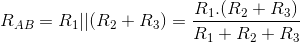Now, one star system is connected to these points A, B, and C as shown in the figure. Three arms RA, RB and RC of the star system are connected with A, B and C respectively. Now if we measure the resistance value between points A and B, we will get,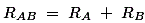Since the two systems are identical, resistance measured between terminals A and B in both systems must be equal.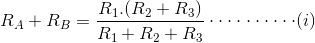Similarly, resistance between points B and C being equal in the two systems,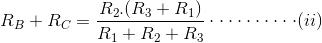And resistance between points C and A being equal in the two systems,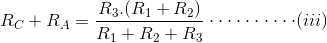Adding equations (I), (II) and (III) we get,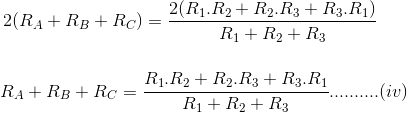Subtracting equations (I), (II) and (III) from equation (IV) we get,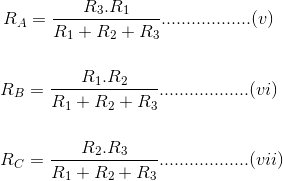The relation of delta - star transformation can be expressed as follows.
The equivalent star resistance connected to a given terminal, is equal to the product of the two delta resistances connected to the same terminal divided by the sum of the delta connected resistances.
If the delta connected system has same resistance R at its three sides then equivalent star resistance r will be,

## Delta – Star Example:

Convert the following Delta Resistive Network into an equivalent Star Network.## Star - Delta Transformation

For star - delta transformation we just multiply equations (v), (VI) and (VI), (VII) and (VII), (V) that is by doing (v) × (VI) + (VI) × (VII) + (VII) × (V) we get,Now dividing equation (VIII) by equations (V), (VI) and equations (VII) separately we get,## Star – Delta Example

Convert the following Star Resistive Network into an equivalent Delta Network.Both Star Delta Transformation and Delta Star Transformation allows us to convert one type of circuit connection into another type in order for us to easily analyse the circuit. These transformation techniques can be used to good effect for either star or delta circuits containing resistances or impedances.

sources: electrical4u.com, electronics-tutorials.ws#### COMMENTS

Name

BASIC ELECTRICAL,12,BATTERIES,4,CIRCUIT THEORIES,9,CONTROL SYSTEMS,3,DC MOTOR,1,DIGITAL ELECTRONICS,1,DISTRIBUTED GENERATION,2,DISTRIBUTION,6,ELECTRICAL DRIVES,1,ELECTRICAL LAWS,8,ELECTRONICS DEVICES,2,General,7,GENERATION,3,GENERATOR,1,HIGH VOLTAGE,4,ILLUMINATION,1,INDUCTION MOTOR,7,MATERIALS,1,MEASUREMENT,1,MOTOR,1,POWER ELECTRONICS,2,PROJECTS ON INDUCTION MOTOR,1,PROTECTION,1,SMART GRID,3,SWITCHGEAR,4,SYNCHRONOUS MOTOR,1,TRANSFORMER,6,TRANSMISSION,4,
ltr
item
Electrical for Us: Star Delta Transformation
Star Delta Transformation
https://4.bp.blogspot.com/-USdm5hUVAdY/WFAxXzNsISI/AAAAAAAAAjI/pK76iSy-TYIG73Jo9MmWmnnQhSQ9EBrtgCLcB/s320/QRk8i.jpg
https://4.bp.blogspot.com/-USdm5hUVAdY/WFAxXzNsISI/AAAAAAAAAjI/pK76iSy-TYIG73Jo9MmWmnnQhSQ9EBrtgCLcB/s72-c/QRk8i.jpg
Electrical for Us
https://www.sanjaysah.com.np/2016/12/star-delta-transformation.html
https://www.sanjaysah.com.np/
http://www.sanjaysah.com.np/
http://www.sanjaysah.com.np/2016/12/star-delta-transformation.html
true
3851448774078769448
UTF-8